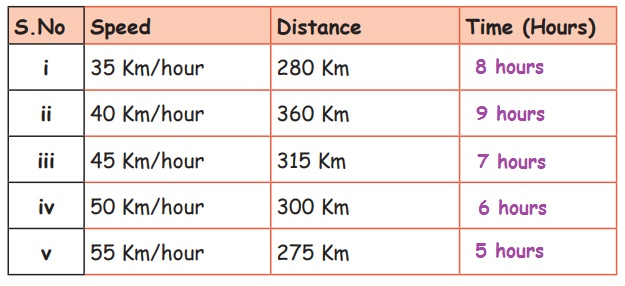Home | | Maths 5th Std | Exercise 5.2 (Speed, time and Distance)

# Exercise 5.2 (Speed, time and Distance)

Text Book Back Exercises Questions with Answers, Solution : 5th Maths : Term 2 Unit 5 : Interconcept : Exercise 5.2 : Relation between Speed, time and Distance

Exercise 5.2i) Speed = 35 km/hour

Distance = 280 kmTime = Distance / Speed = 280/35 = 8 hours

ii) Speed = 40 km / hour

Distance = 360 kmTime = Distance/Speed = 360/40 = 9 hours

iii) Speed = 45 km/hour

Distance = 315 kmTime = Distance/Speed = 315/45 = 7 hours

iv) Speed = 50 km/hour

Distance = 300 kmTime = Distance/Speed = 300/50 = 6 hours

v) Speed = 55 km/hour

Distance = 275 kmTime = Distance/Speed = 275/55 = 5 hours

2. Wilson reached 240 Km at the speed of 60 Km/hour. How much time did he travelled?

Distance travelled = 240 km

Speed = 60 km/hourTime = Distance/Speed = 240/60 = 4 hours

Time taken for his travel = 4 hours

3. Anbarasan travelled 350 Km at the speed of 70 Km/hour. Find the time taken for his travel.

Distance travelled = 350 km

Speed = 70 km/hourTime = Distance/Speed = 350/70 = 5 hours

Time taken for his travel = 5 hours

4. Nazar travelled 360 Km at the speed of 90 Km/hour. Find the time taken for his travel.

Distance travelled = 360 km

Speed = 90 km/hourTime = Distance/Speed = 360/90 = 4 hours

Time taken for his travel = 4 hours

5. Fathima reached 480 Km at the speed of 120 Km/hour. How much time did she travelled?

Distance travelled = 480 km

Speed = 120 km/hourTime = Distance/Speed = 480/120 = 4 hours

Time taken for his travel = 4 hours

Exercise 5.2

1. i) 8 hours ii) 9 hours iii) 7 hours iv) 6 hours v) 5 hours

2. 4 hours

3. 5 hours

4. 4 hours

5. 4 hours

Tags : Interconcept | Term 2 Chapter 5 | 5th Maths , 5th Maths : Term 2 Unit 5 : Interconcept
Study Material, Lecturing Notes, Assignment, Reference, Wiki description explanation, brief detail
5th Maths : Term 2 Unit 5 : Interconcept : Exercise 5.2 (Speed, time and Distance) | Interconcept | Term 2 Chapter 5 | 5th Maths